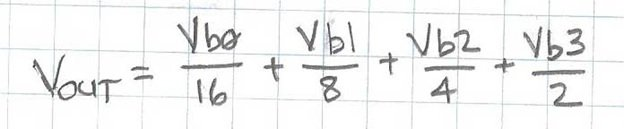# Digital To Analog Converter Introduction and DAC Types

In real Life, Most of the data that we have is Analog in nature. Why do we need converters? We need data converters so that we can convert this analog or digital data available to us in some other form. There are two kinds of data converters present to convert the data, Analog to Digital Converter (ADC) and Digital to Analog converter (DAC).

### Introduction to Digital to analog converter

In the field of electronics Digital to Analog converter is a type of system that is used for the conversion of Digital signals or data into the Analog signals or data. While Analog to Digital converter behaves as a reverse of Digital to Analog converter. During the manipulation of data, the two of the conversion interfaces serves as the backbone to the Digital electronic equipment’s or we can say an analog electronic device. And then these devices needs a processor in order to perform the required operation.

For Example, if we take the illustration of the given below DSP. The Analog to Digital Converter will convert the Analog data like the data that is being collected as the audio input such as Microphone to digital signal that will be processed by the computer. Now after the Conversion from ADC, the digital to analog converter the digital sound signal of the speaker back into analog signal that is useable by the speaker as the output audio equipment.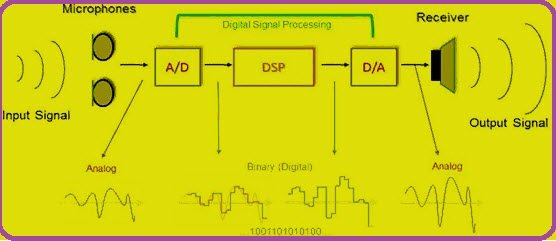### Digital To Analog Converter (DAC) Working

Digital to Analog converter is a system that converts the digital signals into the analog signals. According to the Nyquist-Shannon sampling theory, sampled data can be reconstructed approximately perfectly with a proper bandwidth and the Criteria given by Nyquist.

In a Digital to Analog converter, it is feasible to reconstruct a sampled amount of data into an analog signal with an accurate and effective precision. After this, The amount of digital data can be produced from Application Specific Integrated Systems(ASIC’s), Microprocessors or from a Field Programmable Gate Array(FPGA). This type of data requires an ultimate conversion to the analog signal or to the analog data so that it can interact in the real world. While in the communication systems, Digital transmission of data is a faster and a more convenient way to send data from one place to another, but in all this the digital signals have to be converted into the analog ones. Many of the Digital to Analog converters serves as the sub parts of the Circuitry that is used in the Analog to digital Converters.

### Types Of Digital To Analog Converter

There are mainly two types of methods that are commonly used for digital to analog conversions. The Weighted Resistor method, the R-2R Ladder Network Method along with The Serial Digital to Analog Converter, BCD Digital to Analog Converter and the Bipolar Digital to Analog Converter.

### DAC using Weighted Resistor Method

The weighted resistor method is shown by the following figure.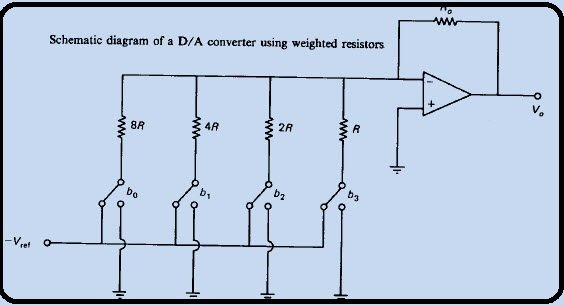This type consist of the following major four components present,

1. N number of switches that represents a bit applied to the input provided.
2. A ladder network supported by a weighted resistor. The resistor is inversely proportional to the corresponding binary digital signal.
3. A reference voltage V ref provided
4. A summing Amplifier

The Circuit that is provided can easily be analyzed by using the “Mill man’s Theorem” that states, “Voltage appearing at any node in a resistive network is equal to the summation of the current entering the node divided by the summation of the conductance connected to the node”

1. The Resistors that are being used in the network have a wide variety of values present that ensures the stability and the absolute accuracy across all the resistors.
2. When the n given by the user is large, the corresponding resistance provided to LBS is considered to be a large value. If it is compared with the provided input resistance of the Amplifier. This all leads to accurate results.

The R-2R electrical device ladder network directly converts a parallel digital voltage into an analog voltage. every digital input (b0, b1, etc.) adds its own weighted contribution to the analog output. This network has some distinctive and attention-grabbing properties. Easily climbable to any desired variety of bits Uses solely 2 values of resistors that work simple and correct fabrication and integration Output resistance is adequate to R, despite the amount of bits, simplifying filtering and additional analog signal process circuit style Analyzing the R-2R network brings back recollections of the ostensibly infinite kind of networks that you’re asked to unravel throughout your undergrad engineering science studies.

### working of  R-2R Ladder Network digital to analog converter

The fact , is that the analysis of this network and the way it works is kind of easy. By organized application of Thevenin Equivalent circuits and Superposition, we will simply show however the R-2R circuit works. Let’s start by analyzing the output resistance. operating through the circuit, simplifying it with Thevenin equivalents, makes this method easy. Thevenin says that if your circuit contains linear components like voltage sources, current sources and resistors, that you just will cut your circuit at any purpose and replace everything on one facet of the cut with a voltage supply and one series electrical device. The voltage supply is that the open-circuit voltage at the cut purpose, and also the series electrical device is that the equivalent circuit resistance with all voltage sources shorted.A pair of below shows the locations of the “cut lines” we’ll use to modify this circuit to calculate its output resistance. For this analysis, the digital inputs can all be thought-about shorted to ground. The two a pair of R resistors to the left of the primary cut line in Figure 2 seem in parallel (when the digital bit b0 is grounded), and may get replaced with one electrical device R as shown in Figure three. The series combination of the 2 R electrical devices on the left of Figure three mix to one resistor valuable 2R, that is in parallel with the 2R electrical device to b1. You may notice that this method repeats itself anytime we have a tendency to work from left to right, in turn substitution mixtures of resistors with their equivalents.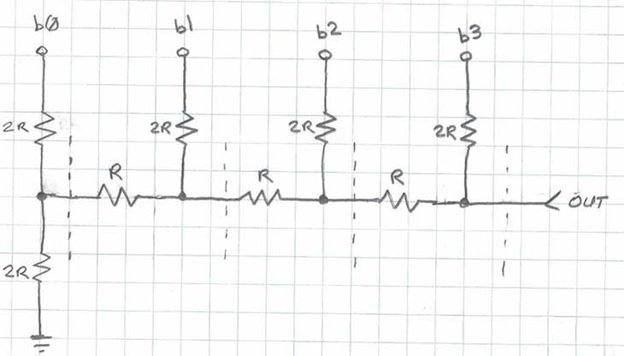As you’ll see in Figure three, the circuit ultimately simplifies to one electrical device R. Thus, the output resistance of the R-2R electrical device network is usually adequate to R, despite the dimensions (number of bits) of the network. This simplifies the planning of any filtering, amplification or extra analog signal acquisition electronic equipment which will follow the network. Next, we’ll examine the way to calculate the analog voltage output for a given parallel digital input on the b0, b1, etc. inputs.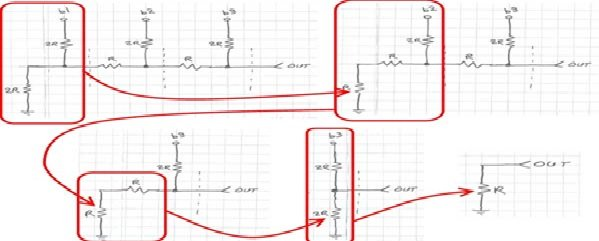We’ll use an equivalent Thevenin equivalents technique shown higher than, further as Superposition. Superposition tells U.S.A. that if you separately calculate the contribution of a given supply to the output (with all others voltage sources shorted and current sources opened), you’ll then total the results for every of the sources to get the ultimate result for the output. We’ll calculate the contribution of 2 of the bits of our 4-bit R-2R DAC in Figure four to point out the method. We’ll assume the bits b0 and b2 area unit logic high, and bits b1 and b3 area unit logic low (ground).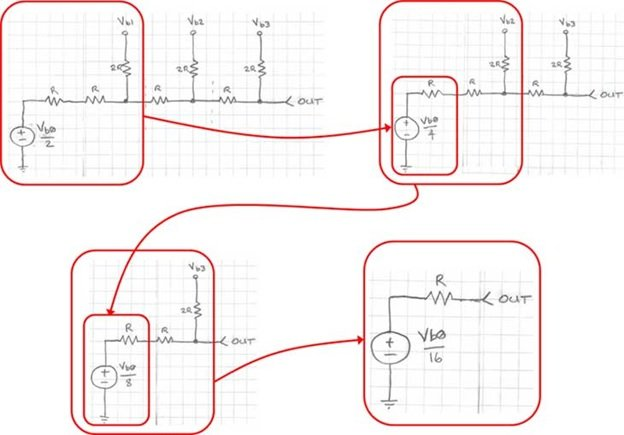From the Thevenin equivalent analysis shown earlier, we all know that we will replace any portion of this circuit to the left of any of the cut lines with a electrical device valuable R, shown because the opening move in Figure five. Next, we have a tendency to follow an equivalent Thevenin equivalent method to the output. As you’ll have already suspected, the contribution of bit b2 is solely Vb2/4. Thus, the analog output voltage once bits b0 and b2 area unit adequate to logic one is solely given by Vb0/16 + Vb2/4. In a a lot of general sense, the contribution of every bit to the output could be a easy binary coefficient operate of every bit. As you’re employed back from the savings bank to the LSB, the voltage contribution every bit is cut in 0.5.